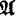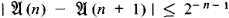# Constructive Mathematics

The following article is from The Great Soviet Encyclopedia (1979). It might be outdated or ideologically biased.

## Constructive Mathematics

the abstract science of constructive processes and their results—constructive objects—and of man’s ability to realize these processes. The nature of the abstractness of constructive mathematics is first and foremost apparent in its systematic use of two abstractions: the abstraction of potential realizability and the abstraction of identification. The former is used whenever we disregard the practical limitations of constructive possibilities in space, time, and matter. The latter abstraction is used when we refer to two objects that are in some sense identical as one and the same object. Constructive mathematics does not make use of the abstraction of actual infinity, which is characteristic of set-theoretic mathematics and views processes that are never completed as indefinitely continued and thereby somehow completed.

A constructive process, the result of which is an object identical to A, is called the construction of the object A. Statements associated with man’s ability to carry out constructive processes are often formulated in constructive mathematics in the form of existence theorems asserting that there exists an object satisfying certain requirements. What is meant here is that the construction of this object is potentially realizable—that is, we possess methods for constructing it. This view of existence theorems differs from its counterpart in set-theoretic mathematics. As a result, we are forced to construct a logic for constructive mathematics that differs from the classical mathematical logic serving set-theoretic mathematics. This logic is called constructive mathematical logic.

The concepts of constructive process and constructive object are not defined in constructive mathematics. There is no need for such general definitions, since in constructive mathematics we do not ordinarily deal with constructive processes or constructive objects in general but rather with specific types of one or the other.

The simplest constructive objects are words in a specified alphabet—that is, sequences of letters in this alphabet (the word “letter” is understood here as an “elementary symbol,” that is, a “symbol whose parts we are not interested in”; an alphabet is simply a set of letters). The constructive process, the result of which is a word, consists in writing down this word letter by letter. Natural numbers are a particular case of words; we define natural numbers as words in the alphabet 01 beginning with zero and otherwise not containing zero—that is, as the words 0, 01, 011, 0111, … . The addition of the minus sign – and the fraction sign / to this alphabet makes it possible to construct the rational numbers as certain words in the alphabet 01 – /. Thus, the rational numbers prove to be constructive objects.

Naturally, there is the task of constructing the real numbers within the framework of constructive mathematics and of including in it mathematical analysis. This has been achieved on the basis of a more precise concept of an algorithm. It is immaterial which of the well-known refinements of this concept is used (Turing machine, recursive functions, normal algorithms). In what follows, the term “algorithm” is understood to mean normal algorithm.

An algorithm that transforms every natural number into a rational (natural) number will be called a constructive sequence of rational (natural) numbers. Without significant loss of generality, we may consider a constructive sequence of rational numbers as an algorithm in the alphabet 01 – /ab. This algorithm will be written as a word in the alphabet 01. We say that a constructive sequence of rational numbers( converges regularly if for every natural number n the following condition holds:Recorded regularly converging sequences of rational numbers are called constructive real numbers. Equality and order between constructive real numbers, as well as arithmetic operations on them and the operation of taking the absolute value, are defined in a natural way. Arithmetic operations prove to be algorithmic. For example, there exists an algorithm that transforms every pair of constructive real numbers into their sum. On the other hand, there exists no algorithm that would recognize that a word in the alphabet 01 is a constructive real number and no algorithm that would recognize the equality of two constructive real numbers.

Furthermore, on the basis of the algorithms of the theory, it is possible to define the concept of a constructive sequence of constructive real numbers. For every such sequence it proves possible to construct a constructive real number that is not equal to any member of the sequence. This is the constructive analog of Cantor’s theorem of the nondenumerability of the continuum.

It is possible to define a constructive Cauchy sequence of constructive real numbers and the convergence of a constructive sequence of constructive real numbers to a constructive real number. The completeness theorem asserting that every constructive Cauchy sequence of constructive real numbers constructively converges to some constructive real number holds.

However, the constructive analog of the well-known convergence theorem for a bounded increasing sequence is refuted by an example.

By definition, a constructive real number is a word in the alphabet 01. The algorithms in this alphabet can be applied to constructive real numbers, which allows us to construct a function of a real variable as an algorithm that transforms constructive real numbers into constructive real numbers. It is only necessary that this algorithm be consistent with equality—that is, it must transform equal constructive real numbers into equal constructive real numbers. Thus, we have the following definition: An algorithm F in the alphabet 01 is a constructive function of a real variable if these conditions are satisfied: (1) F transforms every constructive real number to which it is applied into a constructive real number and (2) each time F is applicable to some constructive real number x it is also applicable to every constructive real number x equal to x, and the constructive real numbers F(x) and F(y) are equal.

A constructive theory of functions of a real variable has been developed on the basis of this definition. One of its most interesting results is the theorem of the continuity of constructive functions, which states that every constructive function of a real variable is continuous wherever it is defined. At the same time it has been proved that the analogs of Weierstrass’ and Cantor’s theorems of continuous functions on a closed interval do not hold in the theory of constructive functions. In particular, the following have been constructed: (1) an unbounded constructive (and therefore continuous) function on the interval [0, 1], (2) a constructive function bounded on this interval and not having a least upper bound, (3) a constructive function that has a least upper bound on the interval [0, 1] but does not attain it, and (4) a constructive function bounded on the interval [0, 1] that is not uniformly continuous on any subinterval of the interval [0, 1]. These results reveal the profound difference between constructive mathematical analysis and set-theoretic analysis.

Many branches of constructive mathematics are being developed at the present time (1970’s). Among them are constructive theories of differentiation and integration, the constructive theory of metric spaces, constructive functional analysis, and the constructive theory of functions of a complex variable.

### REFERENCES

Markov, A. A. “Teoriia algorifmov.” Tr. Matematicheskogo in-ta AN SSSR, 1954, vol. 42.
”Problemy konstruktivnogo napravleniia-matematike,” fascs. 1–5. Ibid, 1958, vol. 52; 1962, vol. 67; 1964, vol. 72; 1967, vol. 93; 1970, vol. 113.
Phan Dinh Dieu. “Nekotorye voprosy konstruktivnogo funktsional’nogo analiza.” Tr. Matematicheskogo in-ta AN SSSR, 1970, vol. 114.

A. A. MARKOV

References in periodicals archive ?
As a variety of constructive mathematics, intuitionism is essentially a philosophy of the foundations of mathematics.
Constructive mathematics is claimed to be a subset of classical mathematics.
(4) In constructive mathematics, for any real number x we can not prove that x [less than or equal to] 0 or x > 0, that x > 0 or x = 0 or x < 0.
These types of qualitative models are all instruments for thinking--the achievement of practical results based on systematic and generalized experiences--and they correspond closely to the concepts of constructive mathematics. An algorithm is the entire set of rules that determine the development of the objects to be constructed.
Borwein (experimental and constructive mathematics, Simon Fraser U.) and Devlin (language and information, Stanford U.) give newcomers to experimental mathematics a short but rigorous introduction to current theories and practices in experimental mathematics.
For the most part, constructive mathematics in the Brouwerian tradition has been pursued as pure mathematics, with little concern for applications in the sciences (with the obvious exception of 'computer science', at least on the part of constructivists accepting Church's Thesis).
Since the seminal work of Errett Bishop , it has been clear that, in fact, constructive mathematics is surprisingly rich in its capacity to develop serviceable constructive versions of classical mathematical theories central in scientific applications, including the theory of metric spaces, Banach and Hilbert spaces, and a good theory of measure.
For example, he seems in some passages (e.g., in 1947) to suggest that in constructive mathematics the objects are completely given, or they can be intuited individually, while this is not the case in higher, impredicative set theory.
On a purely mathematical register, there is much to recommend situating intuitionism within the constructivist orientation, and it is common practice in the literature to use the expressions 'constructive mathematics' and 'intuitionist mathematics' more or less interchange ably.
Material is arranged in chapters on predicate logic, axiomatic set theory, recursion theory and computability, Godel's incompleteness theorems, model theory, contemporary set theory, nonstandard analysis, and constructive mathematics. Appendices supply some background materials.

Site: Follow: Share:
Open / Close